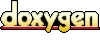# ACE_Sample_History Class Reference

Save multiple samples in an array. More...

`#include <Sample_History.h>`

List of all members.

## Public Member Functions

ACE_Sample_History (size_t max_samples)
Constructor.

~ACE_Sample_History (void)
Destructor.

int sample (ACE_UINT64 value)
Record one sample.

size_t max_samples (void) const
Returns the maximum number of samples.

size_t sample_count (void) const
Returns the current number of samples.

void dump_samples (const ACE_TCHAR *msg, ACE_UINT32 scale_factor) const
Dump all the samples.

void collect_basic_stats (ACE_Basic_Stats &) const
Collect the summary for all the samples.

ACE_UINT64 get_sample (size_t i) const
Get a sample.

## Private Attributes

size_t max_samples_
The maximum number of samples.

size_t sample_count_
The current number of samples.

ACE_UINT64samples_
The samples.

## Detailed Description

Save multiple samples in an array.

Save multiple samples (usually latency numbers), into an array, and later print them in several formats.

Definition at line 34 of file Sample_History.h.

## Constructor & Destructor Documentation

 ACE_BEGIN_VERSIONED_NAMESPACE_DECL ACE_Sample_History::ACE_Sample_History ( size_t max_samples )
 Constructor. The number of samples is pre-allocated, and cannot changes once the class is initialized. Definition at line 18 of file Sample_History.cpp. References ACE_NEW, and ACE_UINT64. ```00019 : max_samples_ (max_samples) 00020 , sample_count_ (0) 00021 { 00022 ACE_NEW(this->samples_, ACE_UINT64[this->max_samples_]); 00023 } ```

 ACE_Sample_History::~ACE_Sample_History ( void )
 Destructor. Definition at line 25 of file Sample_History.cpp. References samples_. ```00026 { 00027 delete[] this->samples_; 00028 } ```

## Member Function Documentation

 void ACE_Sample_History::collect_basic_stats ( ACE_Basic_Stats & ) const
 Collect the summary for all the samples. Definition at line 57 of file Sample_History.cpp. References ACE_Basic_Stats::sample(), and sample_count_. ```00058 { 00059 for (size_t i = 0; i != this->sample_count_; ++i) 00060 { 00061 stats.sample (this->samples_[i]); 00062 } 00063 } ```

 void ACE_Sample_History::dump_samples ( const ACE_TCHAR * msg, ACE_UINT32 scale_factor ) const
 Dump all the samples. Prints out all the samples, using msg as a prefix for each message. Definition at line 43 of file Sample_History.cpp. References ACE_CU64_TO_CU32, ACE_DEBUG, ACE_LIB_TEXT, ACE_TCHAR, ACE_UINT64, LM_DEBUG, sample_count_, and samples_. ```00045 { 00046 #ifndef ACE_NLOGGING 00047 for (size_t i = 0; i != this->sample_count_; ++i) 00048 { 00049 const ACE_UINT64 x = this->samples_[i] / scale_factor; 00050 const ACE_UINT32 val = ACE_CU64_TO_CU32 (x); 00051 ACE_DEBUG ((LM_DEBUG, ACE_LIB_TEXT("%s: %d %u\n"), msg, i, val)); 00052 } 00053 #endif /* ACE_NLOGGING */ 00054 } ```

 ACE_INLINE ACE_UINT64 ACE_Sample_History::get_sample ( size_t i ) const
 Get a sample. Definition at line 18 of file Sample_History.inl. References sample_count_, and samples_. ```00019 { 00020 if (this->sample_count_ <= i) 00021 return 0; 00022 00023 return this->samples_[i]; 00024 } ```

 size_t ACE_Sample_History::max_samples ( void ) const
 Returns the maximum number of samples. Definition at line 31 of file Sample_History.cpp. References max_samples_. ```00032 { 00033 return this->max_samples_; 00034 } ```

 ACE_BEGIN_VERSIONED_NAMESPACE_DECL ACE_INLINE int ACE_Sample_History::sample ( ACE_UINT64 value )
 Record one sample. Return 0 on success, -1 if the sample could not be stored Definition at line 8 of file Sample_History.inl. References ACE_UINT64, max_samples_, sample_count_, and samples_. ```00009 { 00010 if (this->sample_count_ >= this->max_samples_) 00011 return -1; 00012 00013 this->samples_[this->sample_count_++] = value; 00014 return 0; 00015 } ```

 size_t ACE_Sample_History::sample_count ( void ) const
 Returns the current number of samples. Definition at line 37 of file Sample_History.cpp. References sample_count_. ```00038 { 00039 return this->sample_count_; 00040 } ```

## Member Data Documentation

 size_t ACE_Sample_History::max_samples_` [private]`
 The maximum number of samples. Definition at line 75 of file Sample_History.h. Referenced by max_samples(), and sample().

 size_t ACE_Sample_History::sample_count_` [private]`
 The current number of samples. Definition at line 78 of file Sample_History.h. Referenced by collect_basic_stats(), dump_samples(), get_sample(), sample(), and sample_count().

 ACE_UINT64* ACE_Sample_History::samples_` [private]`
 The samples. Definition at line 81 of file Sample_History.h. Referenced by dump_samples(), get_sample(), sample(), and ~ACE_Sample_History().

The documentation for this class was generated from the following files:
Generated on Thu Nov 9 11:28:25 2006 for ACE by1.3.6